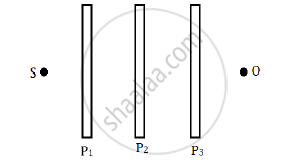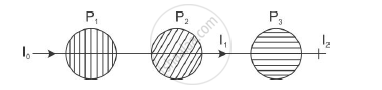# Three Identical Polaroid Sheets P1, P2 and P3 Are Oriented So that the Pass Axis of P2 and P3 Are Inclined at Angles of 60° and 90° Respectively with the Pass Axis of P1. - Physics

Three identical polaroid sheets P1, P2 and P3 are oriented so that the pass axis of P2 and P3 are inclined at angles of 60° and 90° respectively with the pass axis of P1. A monochromatic source S of unpolarised light of intensity I0 is kept in front of the polaroid sheet P1 as shown in the figure. Determine the intensities of light as observed by the observer at O, when polaroid P3 is rotated with respect to P2 at angles θ = 30° and 60°.#### SolutionThe ray of light passing through polaroid P1 will have intensity reduced by half.'

I_1=I_0/2

Now, the polaroid P2 is oriented at an angle 60° with respect to P1.
Therefore, the intensity is

I_2=I_1cos^2 60=I_0/2xx1/4=I_0/8

Now, the polaroid P3 is originally oriented at an angle 90 − 60 = 30°. Hence, when P3 is rotated by 30°, the angle between P2 and P3 is 60°. Therefore, the intensity is

I_3=I_2cos^2 60=I_0/8cos^2 60=I_0/8xx1/4=I+0/32

Similarly, when P3 is rotated by 60°, the angle between P2 and P3 is 90°.

Therefore, the intensity is I_3=I_2cos^2 90=I_0/8xx0xx0

Concept: Polarisation
Is there an error in this question or solution?
2015-2016 (March) All India Set 3 N

Share